## gain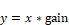Block Category: Arithmetic

Inputs: Real or complex scalars, vectors, or matrices

Description: The gain block multiplies the input signal by the gain amount. You can enter a value in the dialog box; you can alternatively double-click the gain block to enter a value.Gain: Indicates the constant multiplier of the input signal. The default is 1. You can enter a value as a C expression|topic=Entering C expressions, matrix expression, or complex number.

Label: Indicates a user-defined block label that appears when View > Block Labels is activated.

#### Examples

1. Gain of a scalar

Consider the equation y(t) = 3 sin(t), which can be realized as: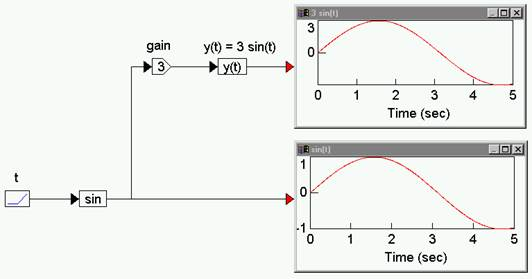A ramp block is used to access simulation time t, a sin block generates sin(t), a gain block amplifies sin(t) to 3 sin(t). Both sin(t) and y(t) are shown in plot blocks for comparison.

2. Gain of a vector

Consider the equation:

z = 7 x

where x = [-1  5.6  4]. This equation can be realized as: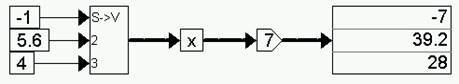The gain block performs an element-by-element gain operation on the incoming vector.

3. Gain of a matrix

Consider the equation:

Z =  4.2 X

where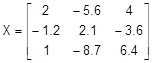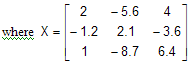This equation can be realized as: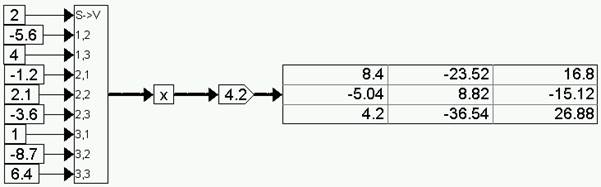The gain block performs an element-by-element gain operation on the incoming matrix.